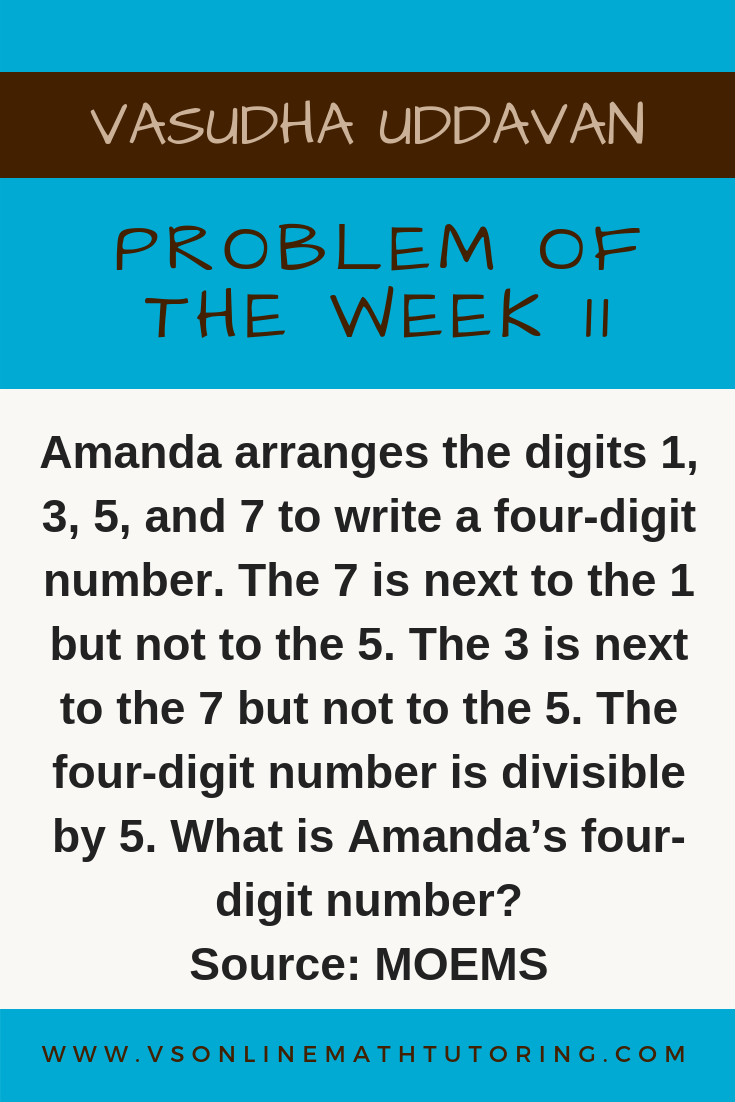Search

# Math Competition Problems - Problem of the Week # 11

Updated: Jan 22, 2019

Amanda arranges the digits 1, 3, 5, and 7 to write a four-digit number. The 7 is next to the 1 but not to the 5. The 3 is next to the 7 but not to the 5. The four-digit number is divisible by 5. What is Amanda’s four-digit number?

Source : MOEMS (Math Olympiad for Elementary and Middle Schools)Math Olympiad Problem - Problem of the week #11

Amanda arranges the digits 1, 3, 5, and 7 to write a four-digit number. The 7 is next to the 1 but not to the 5. The 3 is next to the 7 but not to the 5. The four-digit number is divisible by 5. What is Amanda’s four-digit number?

First clue - The 4 digit number is divisible by 5. Hence the unit's digit must be 5. So our number looks like _ _ _ 5.

Second clue has 2 parts.

Second clue part 1 - The 7 is not next to the 5. So we know that there should be a number between 7 and 5. Which makes our number look like _ 7 _ 5 or 7 _ _ 5.

Second clue part 2 - The 7 is next to the 1

The third clue also has 2 parts.

Third clue part 1- The 3 is next to the 7

Third clue part 2 - The 3 is not next to the 5

Combining Second clue part 2 and Third clue part 1 we get that 7 has 2 numbers adjacent to it. Both 3 an 1 are adjacent to 7. So our number should look like _ 7 _ 5.

Now our third clue says "The 3 is next to the 7 but not to the 5". Hence the 3 has to be in the thousand's place. Our number now is 37_5.

Which means the one has to be in the ten's place. Amanda's number is 3715. Hooray!

This is one of the easier problems from MOEMS. All my 5th graders were able to solve this. The key is to make them write down all the clues and solve it. After finishing I always ask them to check if they've covered all the conditions. Being systematic definitely is needed.

Did you like the problem? Would you like to have these problems and solutions delivered to your inbox as I publish them? Click here to subscribe